## Introduction

Bchron is an R package that enables quick calibration of radiocarbon dates under various calibration curves (including user generated ones); age-depth modelling as per the algorithm of Haslett and Parnell (2008); Relative sea level rate estimation incorporating time uncertainty in polynomial regression models (Parnell and Gehrels 2015); and non-parametric phase modelling via Gaussian mixtures as a means to determine the activity of a site (and as an alternative to the Oxcal function SUM; currently unpublished).

You will find Bchron far easier to use if you know some basics on how to use R. I recommend the book by Norman Matloff amazon.co.uk link, or the online intro course by Code School.

If you find bugs or want to suggest new features please visit the Bchron GitHub issues page.

## Installing Bchron

Bchron will run in Windows, Mac OS X or Linux. To install Bchron you first need to install R. I would also recommend installing Rstudio as a nice desktop environment for using R. Once in R you can type:

``````install.packages('Bchron')
``````

at the R command prompt to install Bchron. If you then type:

``````library(Bchron)
``````
``````## Loading required package: inline
``````

it will load in all the Bchron functions.

Bchron will calibrate single or multiple dates under multiple (even user defined) calibration curves. By default, the intcal13, shcal13 and marine13 calibration curves are included. You can calibrate a single radiocarbon date with, e.g.

``````ages1 = BchronCalibrate(ages=11553,
ageSds=230,
calCurves='intcal13',
ids='Date-1')
summary(ages1)
``````
``````## Highest density regions for Date-1
##        [,1]    [,2]  [,3]    [,4]
## 99% 12808.8 14010.0    NA      NA
## 95% 12953.0 13862.0 13888 13928.7
## 50% 13254.3 13548.1    NA      NA
``````
``````plot(ages1)
``````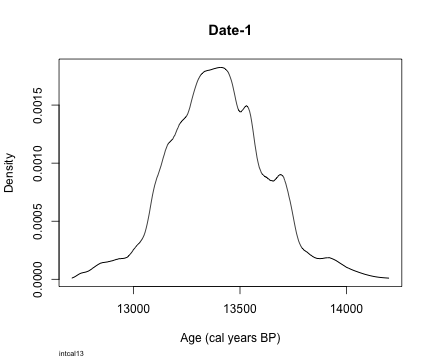This will calibrate the radiocarbon age of 11,553 14C years BP with standard error 230 14C years on the intcal13 calibration curve. The `id` given is optional and only used for summarising and plotting. The summary command then gives the highest density regions of the calibrated date and the plot command produces a simple plot of the density.

Bchron can calibrate multiple dates simultaneously by inputting the dates as vectors:

``````ages2 = BchronCalibrate(ages=c(3445,11553,7456),
ageSds=c(50,230,110),
calCurves=c('intcal13','intcal13','shcal13'))
summary(ages2)
plot(ages2)
``````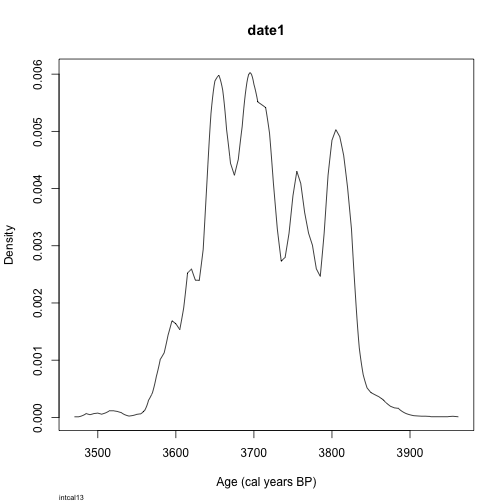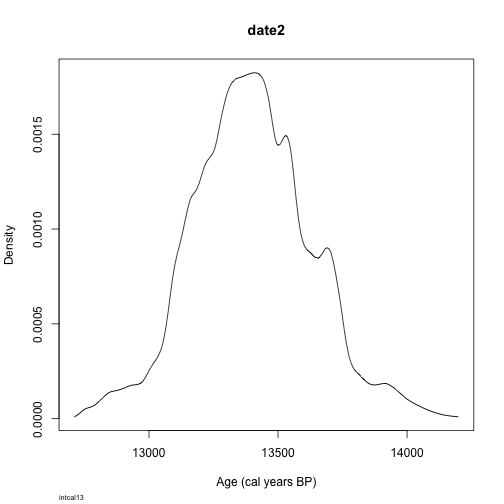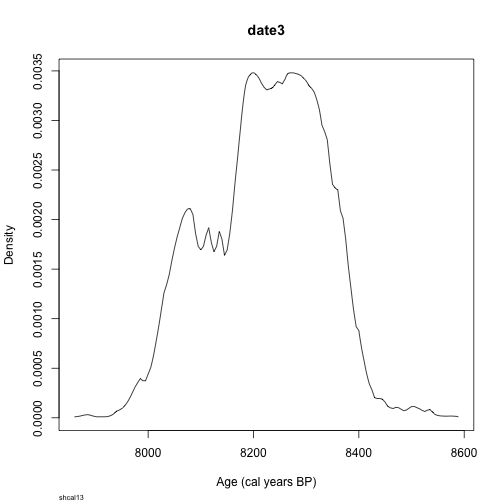This will calibrate three different 14C ages with the calibration curves as specified in the `calCurves` argument. The `summary` and `plot` commands will produce individual highest density regions and density plots for the three dates.

Finally, if you provide position information (e.g. depths) to the BchronCalibrate function it will create a plot with position on the y-axis, e.g.:

``````ages3 = BchronCalibrate(ages=c(3445,11553),
ageSds=c(50,230),
positions=c(100,150),
calCurves=c('intcal13','normal'))
summary(ages3)
``````
``````## Highest density regions for date1
##       [,1]   [,2]   [,3]   [,4]   [,5]   [,6]
## 99% 3563.9 3870.0     NA     NA     NA     NA
## 95% 3590.0 3833.6     NA     NA     NA     NA
## 50% 3641.0 3670.7 3678.2 3723.2 3796.4 3816.5
##
## Highest density regions for date2
##        [,1]    [,2]
## 99% 10973.0 12133.0
## 95% 11099.0 12007.0
## 50% 11402.5 11703.5
``````
``````plot(ages3,withPositions=TRUE)
``````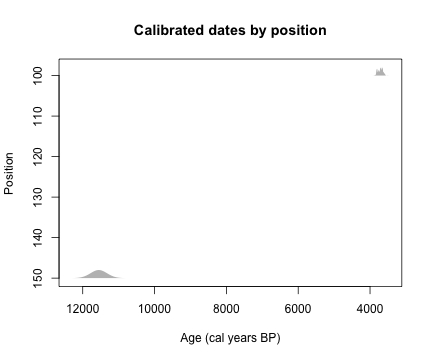The calibration code is very fast. On standard PC you should have no trouble calibrating thousands of dates simultaneously without a noticeably long wait.

## Running the Bchronology age-depth model

The Bchronology function fits the age-depth model outlined by Haslett and Parnell (2008). An illustrative data set from Glendalough is provided with the package, containing 5 radiocarbon dates and a known age for the top of the core. It can be called in via:

``````data(Glendalough)
print(Glendalough)
``````
``````##            id  ages ageSds position thickness calCurves
## 1       Top-1     0      1        0         0    normal
## 2 Beta-122061  2310     60      426         4  intcal13
## 3 Beta-100901  9150     50     1166         4  intcal13
## 4 Beta-100900  9810     60     1204         4  intcal13
## 5 Beta-100899 10940     60     1383         2  intcal13
## 6 Beta-100897 11550     60     1433         2  intcal13
``````

The top date is from the present and has the calibration curve 'normal' as it is not a 14C date. This core can be run through Bchron via:

``````GlenOut = Bchronology(ages=Glendalough\$ages,
ageSds=Glendalough\$ageSds,
calCurves=Glendalough\$calCurves,
positions=Glendalough\$position,
positionThicknesses=Glendalough\$thickness,
ids=Glendalough\$id,
predictPositions=seq(0,1500,by=10))
``````

There are other arguments you can supply to Bchronology, including the date the core was extracted, the outlier probabilities for each individual date, and the number of iterations for which to run the algorithm. For more details see:

``````help(Bchronology)
``````

Once run, the summary commands will show various output:

``````summary(GlenOut)
``````
``````summary(GlenOut, type='convergence')
``````
``````## Convergence check (watch for too many small p-values):
##             p-value
## Outlier 4   0.01785
## Outlier 6   0.01804
## Top-1       0.08465
## RateMean    0.10672
## Outlier 1   0.14683
## Beta-122061 0.24661
## Beta-100901 0.29368
## Beta-100899 0.29948
## Beta-100897 0.35459
## Beta-100900 0.39254
## Outlier 3   0.42872
## Outlier 2   0.43028
## RateVar     0.49596
## Outlier 5   0.49743
``````
``````summary(GlenOut, type='outliers')
``````
``````## Posterior outlier probability by date:
##         Date OutlierProb
##        Top-1       0.004
##  Beta-122061       0.008
##  Beta-100901       0.006
##  Beta-100900       0.007
##  Beta-100899       0.010
##  Beta-100897       0.013
``````

The first summary command produces ages for each position supplied in the `predictPositions` argument above (output not shown as it's too long). The second provides convergence diagnostics. The third gives outlier probabilities. The `plot` command will produce an age-position plot:

``````plot(GlenOut,
main="Glendalough",
xlab='Age (cal years BP)',
ylab='Depth (cm)',
las=1)
``````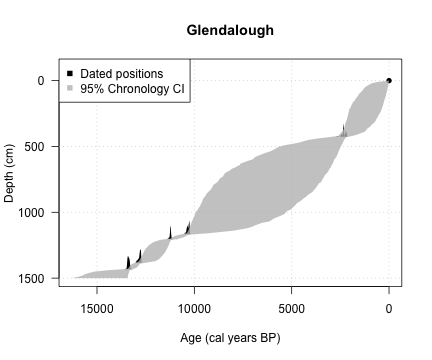Finally, the `predict` command will produce predicted ages for a newly specified set of positions with optional thicknesses:

``````predictAges = predict(GlenOut,
newPositions = c(150,725,1500),
newPositionThicknesses=c(5,0,20))
``````

To run this model on a data set of your own, you will need to load in your data set via, e.g.

``````mydata = read.table(file='path/to/file.txt',header=TRUE)
run = Bchronology(ages=mydata[,1],ageSds=mydata[,2], ...
``````

## Running RSL rate estimation

The function `BchronRSL` will produce estimated relative sea level rates from a regression model taking into account the uncertainties in age provided by a `Bchronology` run as above. Two example data sets are provided:

``````data(TestChronData)
data(TestRSLData)
``````

These can be run through Bchronology and BchronRSL via:

``````RSLrun = Bchronology(ages=TestChronData\$ages,
ageSds=TestChronData\$ageSds,
positions=TestChronData\$position,
positionThicknesses=TestChronData\$thickness,
ids=TestChronData\$id,
calCurves=TestChronData\$calCurves,
predictPositions=TestRSLData\$Depth)
RSLrun2 = BchronRSL(RSLrun,
RSLmean=TestRSLData\$RSL,
RSLsd=TestRSLData\$Sigma,
degree=3)
``````

The `Bchronology` run is as described in the section above. The `BChronRSL` run takes this object, an estimate of the RSL means and standard deviations, and a value of degree (here 3 indicating cubic regression). They can then be summarised and plotted via:

``````summary(RSLrun2)
plot(RSLrun2)
``````

## Running non-parametric phase estimation

Bchron contains two functions for running non-parametric phase models for estimating activity level in a site/region. The first, BchronDensity fits a full Bayesian Gaussian mixture model to the radiocarbon dates whilst the second BchronDensityFast fits an approximate version which will run on much larger data sets. An example run is

``````data(Sluggan)
SlugDens = BchronDensity(ages=Sluggan\$ages,
ageSds=Sluggan\$ageSds,
calCurves=Sluggan\$calCurves,
numMix=50)
``````
``````plot(SlugDens,xlab='Age (cal years BP)')
``````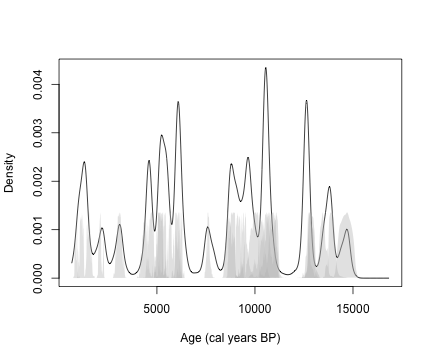BchronDensityFast is identical except for the function call:

``````SlugDensFast = BchronDensityFast(ages=Sluggan\$ages,
ageSds=Sluggan\$ageSds,
calCurves=Sluggan\$calCurves)
``````

## References

For a description of the kind of things that Bchron can do:
Parnell, A. C., Haslett, J., Allen, J. R. M., Buck, C. E., & Huntley, B. (2008). A flexible approach to assessing synchroneity of past events using Bayesian reconstructions of sedimentation history. Quaternary Science Reviews, 27(19-20), 1872–1885.

For the maths behind Bchron:
Haslett, J., & Parnell, A. (2008). A simple monotone process with application to radiocarbon-dated depth chronologies. Journal of the Royal Statistical Society: Series C (Applied Statistics), 57(4), 399–418.

For a review of chronology models:
Parnell, A. C., Buck, C. E., & Doan, T. K. (2011). A review of statistical chronology models for high-resolution, proxy-based Holocene palaeoenvironmental reconstruction. Quaternary Science Reviews, 30(21-22), 2948–2960.

For relative sea level rate estimation:
Parnell, A. C., & Gehrels, W. R. (2015). Using chronological models in late Holocene sea level reconstructions from salt marsh sediments. In: I. Shennan, B.P. Horton, and A.J. Long (eds). Handbook of Sea Level Research. Chichester: Wiley.